# Signal Denoising with Wavelets¶

Important: Please read the installation page for details about how to install the toolboxes. $\newcommand{\dotp}{\langle #1, #2 \rangle}$ $\newcommand{\enscond}{\lbrace #1, #2 \rbrace}$ $\newcommand{\pd}{ \frac{ \partial #1}{\partial #2} }$ $\newcommand{\umin}{\underset{#1}{\min}\;}$ $\newcommand{\umax}{\underset{#1}{\max}\;}$ $\newcommand{\umin}{\underset{#1}{\min}\;}$ $\newcommand{\uargmin}{\underset{#1}{argmin}\;}$ $\newcommand{\norm}{\|#1\|}$ $\newcommand{\abs}{\left|#1\right|}$ $\newcommand{\choice}{ \left\{ \begin{array}{l} #1 \end{array} \right. }$ $\newcommand{\pa}{\left(#1\right)}$ $\newcommand{\diag}{{diag}\left( #1 \right)}$ $\newcommand{\qandq}{\quad\text{and}\quad}$ $\newcommand{\qwhereq}{\quad\text{where}\quad}$ $\newcommand{\qifq}{ \quad \text{if} \quad }$ $\newcommand{\qarrq}{ \quad \Longrightarrow \quad }$ $\newcommand{\ZZ}{\mathbb{Z}}$ $\newcommand{\CC}{\mathbb{C}}$ $\newcommand{\RR}{\mathbb{R}}$ $\newcommand{\EE}{\mathbb{E}}$ $\newcommand{\Zz}{\mathcal{Z}}$ $\newcommand{\Ww}{\mathcal{W}}$ $\newcommand{\Vv}{\mathcal{V}}$ $\newcommand{\Nn}{\mathcal{N}}$ $\newcommand{\NN}{\mathcal{N}}$ $\newcommand{\Hh}{\mathcal{H}}$ $\newcommand{\Bb}{\mathcal{B}}$ $\newcommand{\Ee}{\mathcal{E}}$ $\newcommand{\Cc}{\mathcal{C}}$ $\newcommand{\Gg}{\mathcal{G}}$ $\newcommand{\Ss}{\mathcal{S}}$ $\newcommand{\Pp}{\mathcal{P}}$ $\newcommand{\Ff}{\mathcal{F}}$ $\newcommand{\Xx}{\mathcal{X}}$ $\newcommand{\Mm}{\mathcal{M}}$ $\newcommand{\Ii}{\mathcal{I}}$ $\newcommand{\Dd}{\mathcal{D}}$ $\newcommand{\Ll}{\mathcal{L}}$ $\newcommand{\Tt}{\mathcal{T}}$ $\newcommand{\si}{\sigma}$ $\newcommand{\al}{\alpha}$ $\newcommand{\la}{\lambda}$ $\newcommand{\ga}{\gamma}$ $\newcommand{\Ga}{\Gamma}$ $\newcommand{\La}{\Lambda}$ $\newcommand{\si}{\sigma}$ $\newcommand{\Si}{\Sigma}$ $\newcommand{\be}{\beta}$ $\newcommand{\de}{\delta}$ $\newcommand{\De}{\Delta}$ $\newcommand{\phi}{\varphi}$ $\newcommand{\th}{\theta}$ $\newcommand{\om}{\omega}$ $\newcommand{\Om}{\Omega}$

This tour uses wavelets to perform signal denoising using thresholding estimators. Wavelet thresholding properites were investigated in a series of papers by Donoho and Johnstone, see for instance DonJohn94 and DoJoKePi95.

In :
warning off
warning on


Here we consider a simple additive Gaussian white noise.

Size $N$ of the signal.

In :
N = 2048*2;


First we load the 1-D signal.

In :
name = 'piece-regular';
f0 = rescale(f0,.05,.95);


Variance $\si^2$ of the noise.

In :
sigma = 0.05;


Generate a noisy signal $f = f_0 + w$ where $w \sim \Nn(0,\si^2 \text{Id})$.

In :
f = f0 + randn(size(f0))*sigma;


Display.

In :
clf;
subplot(2,1,1);
plot(f0); axis([1 N 0 1]);
title('Clean signal');
subplot(2,1,2);
plot(f); axis([1 N 0 1]);
title('Noisy signal');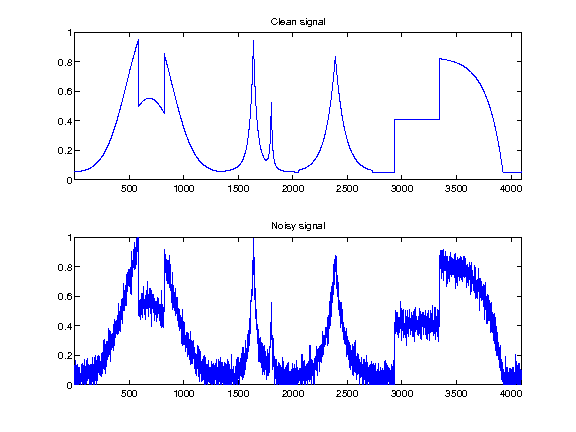## Hard Thresholding vs. Soft Thresholding¶

A thresholding $\Theta : \RR^N \rightarrow \RR^N$ is a non-linear function that operates diagonaly, i.e. $$\forall i, \quad \Theta(x)_i = \th(x_i)$$ where $\th : \RR \rightarrow \RR$ is the 1-D thresholding function.

The most important thresholding are the hard thresholding (related to $\ell^0$ minimization) and the soft thresholding (related to $\ell^1$ minimization).

The hard thresholding reads $$\Theta^0_T(x)_i = \choice{ x_i \qifq \abs{x_i}>T, \\ 0 \quad \text{otherwise}. }$$

In :
Theta0 = @(x,T)x .* (abs(x)>T);


The soft thresholding reads $$\Theta^1_T(x)_i = \max\pa{ 0, 1-\frac{T}{\abs{x}} } x$$

In :
Theta1 = @(x,T)max(0, 1-T./max(abs(x),1e-9)) .* x;


Display the thresholding.

In :
t = linspace(-3,3,1024)'; T = 1;
clf;
plot( t, [Theta0(t,T), Theta1(t,T)], 'LineWidth', 2 );
axis('equal'); axis('tight');
legend('\Theta^0', '\Theta^1');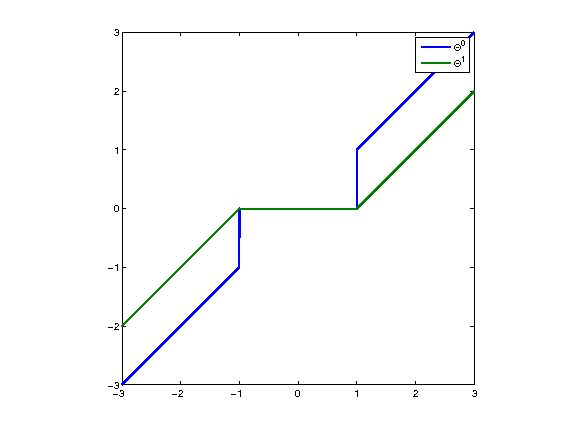## Wavelet Thresholding¶

It is possible to perform non linear denoising by thresholding the wavelet coefficients of $f$.

Shortcut for the foward orthogonal wavelet transform $W$ and the inverse wavelet transform $W^{-1}=W^*$.

In :
options.ti = 0; Jmin = 4;
W  = @(f) perform_wavelet_transf(f,Jmin,+1,options);
Wi = @(fw)perform_wavelet_transf(fw,Jmin,-1,options);


Compute the wavelet coefficients $x=W(f)$.

In :
x = W(f);


Threshold the wavelet coefficients $\tilde x=\Theta_0(x)$. Here we use $T=3\si$.

In :
x1 = Theta0(x, 3*sigma);


Display the wavelet coefficients $W(f)$ and the hard thresholded coefficients $\Theta_0(W(f))$. Note how the wavelet coefficients are contaminated by a small amplitude Gaussian white noise, most of which are removew by thresholding.

In :
clf;
subplot(2,1,1);
plot_wavelet(x,Jmin); axis([1 N -1 1]);
title('W(f)');
subplot(2,1,2);
plot_wavelet(Theta0(W(f),T),Jmin); axis([1 N -1 1]);
title('\Theta_0(W(f))');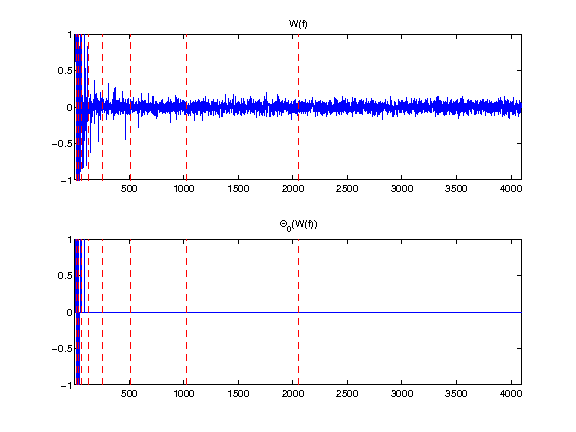Reconstruct from the thresholded coefficients the final estimator $\tilde f = W^*(\tilde x)$.

In :
f1 = Wi(x1);


Display noisy and denoised signal.

In :
clf;
subplot(2,1,1);
plot(f); axis([1 N 0 1]);
title('f');
subplot(2,1,2);
plot(f1); axis([1 N 0 1]);
title('f_1');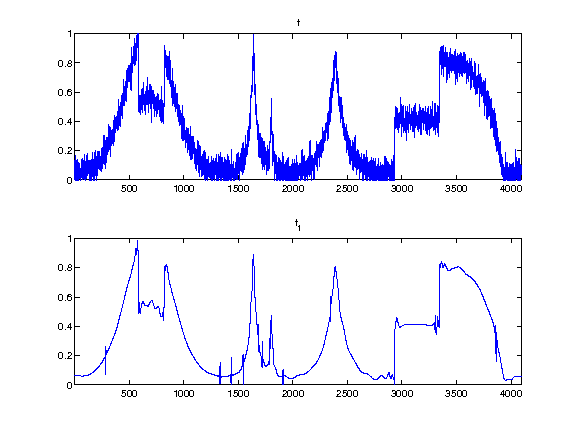Given a thresholding $\Theta$ (for instance $\Theta^0$ or $\Theta^1$), one thus defines a wavelet thresholding estimator as $$\Theta_W(f) = W^* \circ \Theta \circ W.$$

Operator to re-inject the coarse scale noisy coefficients. Improves a little bit the result of soft thresholding denoising (because of the bias).

In :
x = W(f);
reinject = @(x1)assign(x1, 1:2^Jmin, x(1:2^Jmin));


Define the soft and hard thresholding estimators.

In :
Theta0W = @(f,T)Wi(Theta0(W(f),T));
Theta1W = @(f,T)Wi(reinject(Theta1(W(f),T)));


The denoising performance of an estimator $\tilde f = \Theta_W(f)$ is measured using the $L^2$ error to the (unknown) ground trust $f_0$. One usually expresses it in dB using the SNR $$SNR = -10\log_{10}\pa{ \frac{\norm{f_0-\tilde f}^2}{\norm{f_0}^2} }.$$

Exercise 1

Display the evolution of the denoising SNR when $T$ varies. Store in |fBest0| and |fBest1| the optimal denoising results. etrieve best

In :
exo1()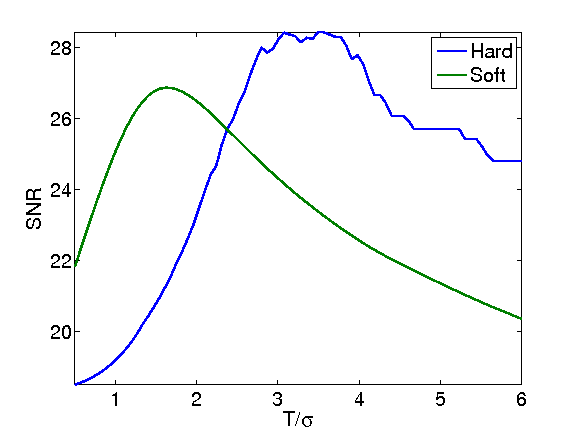In :
%% Insert your code here.


Display the results. For 1-D signals, hard thresholding seems to outperform soft thresholding. For natural images, on contrary, soft thresholding seems to be better.

In :
clf;
subplot(2,1,1);
plot(fBest0); axis([1 N 0 1]); e = snr(fBest0,f0);
title(['Hard, SNR=' num2str(e,3) 'dB']);
subplot(2,1,2);
plot(fBest1); axis([1 N 0 1]); e = snr(fBest1,f0);
title(['Soft, SNR=' num2str(e,3) 'dB']);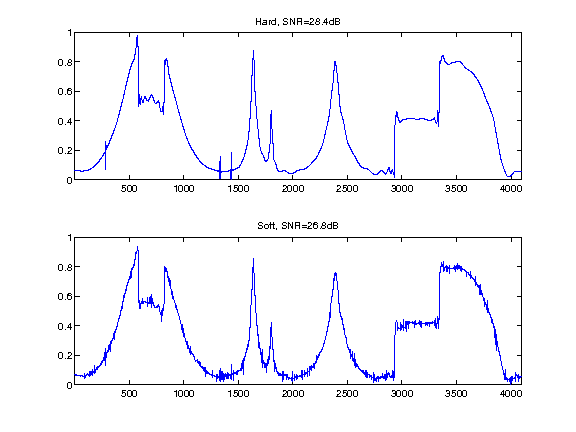## Translation Invariant Wavelet Transform¶

Orthogonal wavelet transforms are not translation invariant. It means that the processing of an image and of a translated version of the image give different results. A translation invariant wavelet transform is implemented by ommitting the sub-sampling at each stage of the transform. This correspond to the decomposition of the image in a redundant familly of $N (J+1)$ atoms where $N$ is the number of samples and $J$ is the number of scales of the transforms.

The foward and backward transform algorithm is the so-called "a trou" algorithm, that was introduced in Holsch87. See also Fowler05 for a review. This algorithm runs in $O(J N)$ operations.

The invariant transform is obtained using the same function, by activating the switch |options.ti=1|.

In :
options.ti = 1;
W  = @(f) perform_wavelet_transf(f,Jmin,+1,options);
Wi = @(fw)perform_wavelet_transf(fw,Jmin,-1,options);


Compute the invariant transform.

In :
fw = W(f);


|fw(:,:,1)| corresponds to the low scale residual. Each |fw(:,1,j)| corresponds to a scale of wavelet coefficient, and has the same size as the original signal.

In :
nJ = size(fw,3)-4;
clf;
subplot(5,1, 1);
plot(f0); axis('tight');
title('Signal');
i = 0;
for j=1:3
i = i+1;
subplot(5,1,i+1);
plot(fw(:,1,nJ-i+1)); axis('tight');
title(strcat(['Scale=' num2str(j)]));
end
subplot(5,1, 5);
plot(fw(:,1,1)); axis('tight');
title('Low scale');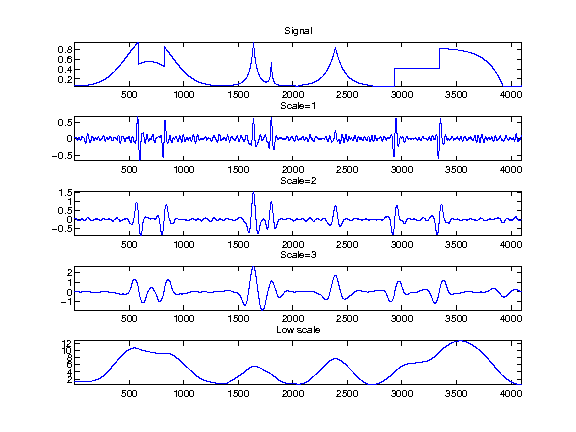## Translation Invariant Wavelet Denoising¶

Orthogonal wavelet denoising does not performs very well because of its lack of translation invariance. A much better result is obtained by not sub-sampling the wavelet transform, which leads to a redundant tight-frame.

Translation invariant denoising using cycle spinning is introduced in CoifDon95. We uwe here the a trou algorithm which is faster.

Operator to re-inject the coarse scales.

In :
x = W(f);
reinject = @(x1)assign(x1, 1:N, x(1:N));


Define the soft and hard thresholding estimators.

In :
Theta0W = @(f,T)Wi(Theta0(W(f),T));
Theta1W = @(f,T)Wi(reinject(Theta1(W(f),T)));


Exercise 2

Display the evolution of the denoising SNR when $T$ varies. Store in |fBest0| and |fBest1| the optimal denoising results. etrieve best

In :
exo2()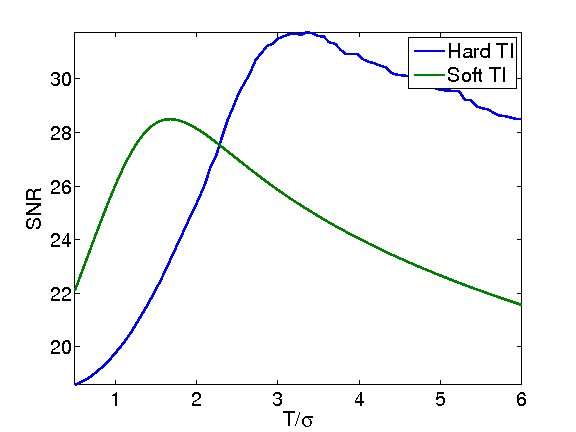In :
%% Insert your code here.


Display the results.

In :
clf;
subplot(2,1,1);
plot(fBest0); axis([1 N 0 1]); e = snr(fBest0,f0);
title(['Hard TI, SNR=' num2str(e,3) 'dB']);
subplot(2,1,2);
plot(fBest1); axis([1 N 0 1]); e = snr(fBest1,f0);
title(['Soft TI, SNR=' num2str(e,3) 'dB']);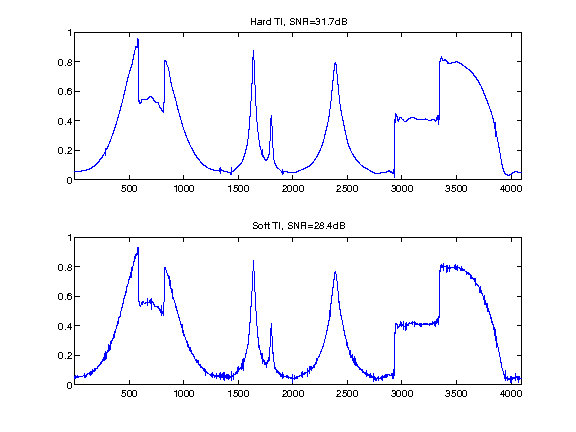## Bibliography¶

• [DonJohn94] D. L. Donoho and I. M. Johnstone, Ideal spatial adaptation via wavelet shrinkage, Biometrika, vol. 81, pp. 425-455, 1994.
• [DoJoKePi95] Donoho, D.L., I.M. Johnstone, G. Kerkyacharian and D. Picard, Wavelet Shrinkage: Asymptopia, J. Roy. Statist. Soc. B 57 2, 301-369,1995.
• [CoifDon95] R.R. Coifman and D.L. Donoho, Translation-Invariant De-Noising, in Wavelets and Statistics, A. Antoniadis and G. Oppenheim, Eds. San Diego, CA: Springer-Verlag, Lecture notes 1995.
• [Fowler05] J. E. Fowler, The redundant discrete wavelet transform and additive noise, IEEE Signal Processing Letters, vol. 12, issue 9, pp. 629-632, 2005.
• [Holsch87] M. Holschneider, R. Kronland-Martinet, J. Morlet, and P. Tchamitchian, A real-time algorithm for signal analysis with the help of the wavelet transform, in Wavelets: Time-Frequency Methods and Phase Space, Springer-Verlag, 1989, pp. 286 297, 1987